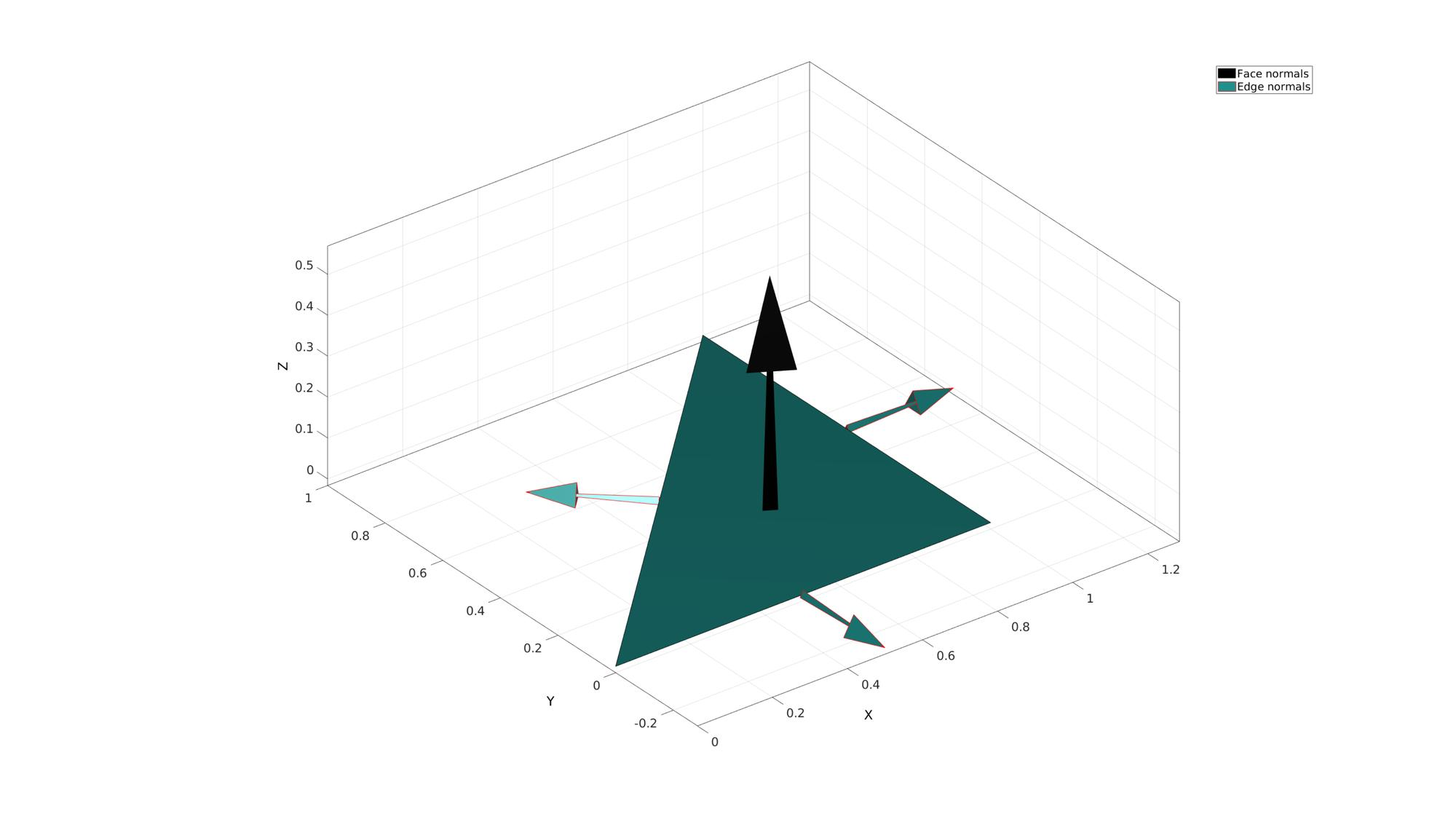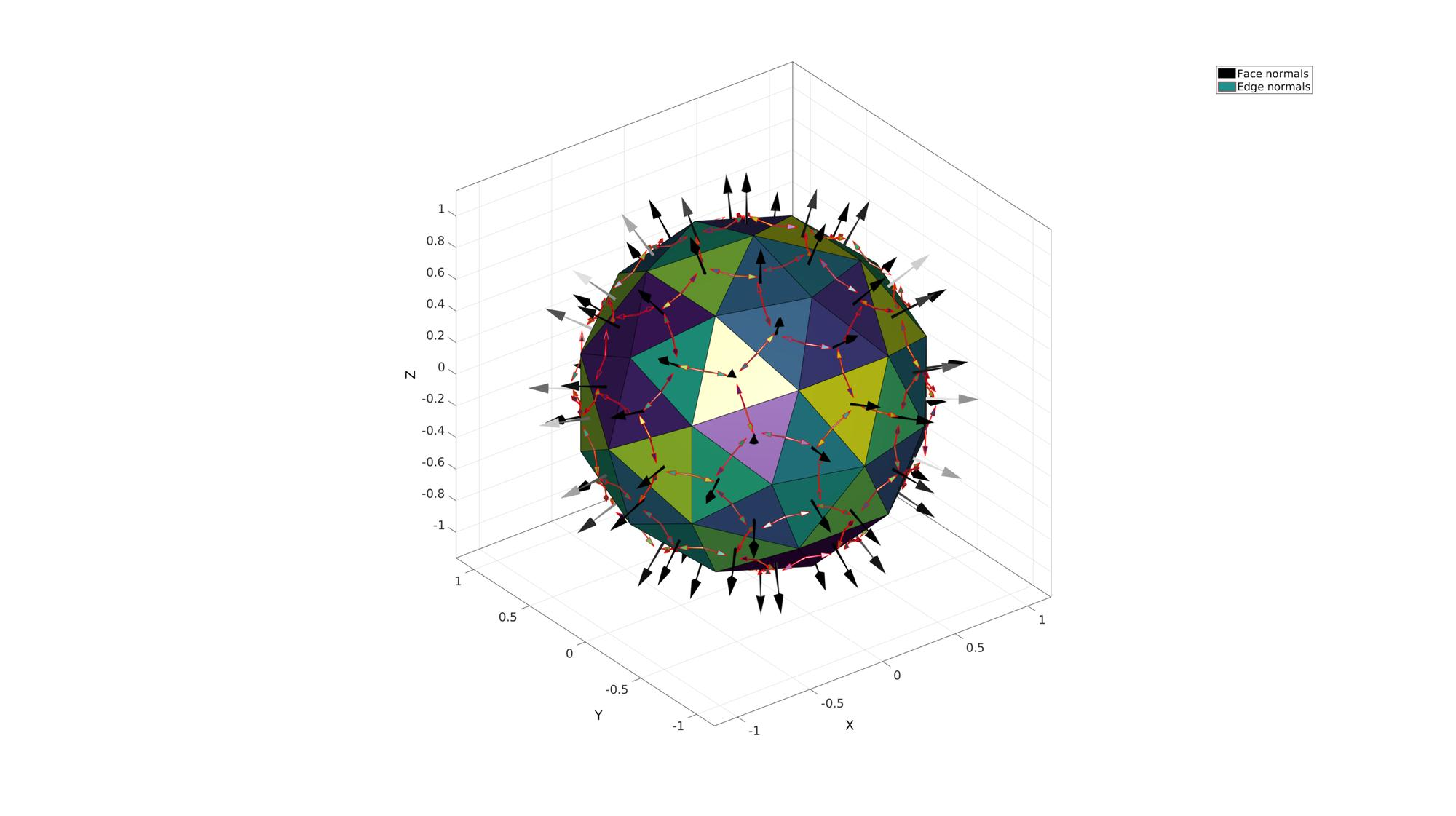# edgeNormal

Below is a demonstration of the features of the edgeNormal function

## Contents

```clear; close all; clc;
```

## Syntax

[NE,VE]=edgeNormal(F,V);

## Description

This function computes the edge normal vectors (NE), and optionally the position vectors for these normal vectors (VE), for the input patch data defined by faces (F) and vertices (V).

## Calculate edge normals for patch data for a single face

Create example patch surface data

```%A single triangle
V=[0 0 0; 1 0 0; 1 1 0];
F=[1 2 3];
```

Get edge normals

```[NE,VE]=edgeNormal(F,V); %Get edge normals
```

Visualize edge normals

```%Use mean edge length devided by 4 as the length for visualizing edge
%vectors.
d=mean(patchEdgeLengths(F,V))/4;

%Create example color data for faces and edges
C=(1:size(F,1))'; %Color data for each face equal to face numbers
CE=[C(:,ones(size(F,2),1))]'; %Get corresponding colors for edges
CE=CE(:);

%Visualize
cFigure;
gpatch(F,V,C,'k');
hp(1)=patchNormPlot(F,V); %Plot face normals
hp(2)=quiverVec(VE,NE,d,CE,'r'); %Plot edge normals
legend(hp,{'Face normals','Edge normals'});
axisGeom;
colormap viridis
drawnow;
```## Calculate edge normals for general patch data

Create example patch surface data

```[F,V]=geoSphere(1,1); %A geodesic sphere triangulation
```

Get edge normals

```[NE,VE]=edgeNormal(F,V); %Get edge normals
```

Visualize edge normals

```%Use mean edge length devided by 4 as the length for visualizing edge
%vectors.
d=mean(patchEdgeLengths(F,V))/4;

%Create example color data for faces and edges
C=(1:size(F,1))'; %Color data for each face equal to face numbers
CE=[C(:,ones(size(F,2),1))]'; %Get corresponding colors for edges
CE=CE(:);

%Visualize
cFigure;
gpatch(F,V,C,'k');
hp(1)=patchNormPlot(F,V); %Plot face normals
hp(2)=quiverVec(VE,NE,d,CE,'r'); %Plot edge normals
legend(hp,{'Face normals','Edge normals'});
axisGeom;
colormap viridis
drawnow;
```GIBBON footer text

GIBBON: The Geometry and Image-based Bioengineering add-On. A toolbox for image segmentation, image-based modeling, meshing, and finite element analysis.

Copyright (C) 2019 Kevin Mattheus Moerman

This program is free software: you can redistribute it and/or modify it under the terms of the GNU General Public License as published by the Free Software Foundation, either version 3 of the License, or (at your option) any later version.

This program is distributed in the hope that it will be useful, but WITHOUT ANY WARRANTY; without even the implied warranty of MERCHANTABILITY or FITNESS FOR A PARTICULAR PURPOSE. See the GNU General Public License for more details.

You should have received a copy of the GNU General Public License along with this program. If not, see http://www.gnu.org/licenses/.